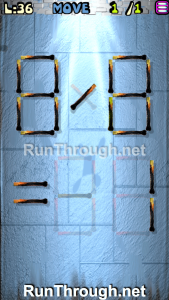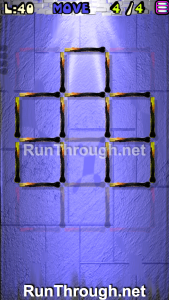# Move the Matches Puzzles Episode 13 Level 21-40 Walkthrough

Walkthrough for all the solutions and answers to the game Move the Matches Puzzles by K-Lab. Get the app on the Google Play Store to play along if you do not already have it. This is for episode 13, levels 21 through 40.

For the walkthrough (or answer) images, we will be showing the final image and answer of the Matches Puzzle. This will allow you to see where everything goes, and to know for sure that the puzzle works.  These puzzles have been recently updated, so other walkthroughs do not have the updated puzzles, but we try to always stay up to date with every walkthrough and answer!

### Level 21Instructions: Move 1 match to correct the equation

Solution: 5-11=-6

### Level 22Instructions: Move 2 matches to correct the equation

Solution: 2+6=8

### Level 23Instructions: Move 2 matches to correct the equation

Solution: 8×7=56

### Level 24Instructions: Remove 4 matches to correct the equation

Solution: 9×5=45

### Level 25Instructions: Move 2 matches to correct the equation

Solution: 9+7=16

### Level 26Instructions: Move 1 match to correct the equation

Solution: 9+4=13

### Level 27Instructions: Remove 4 matches to correct the equation

Solution: 0-11=-11

### Level 28Instructions: Move 1 match to correct the equation

Solution: 9×6=54

### Level 29Instructions: Move 1 match to correct the equation

Solution: 3-6=-3

### Level 30Instructions: Move 3 matches to get 4 squares

### Level 31Instructions: Move 2 matches to correct the equation

Solution: 9×1=09

### Level 32Instructions: Move 3 matches to correct the equation

Solution: 3×3=9

### Level 33Instructions: Move 3 matches to correct the equation

Solution: 5×5=25

### Level 34Instructions: Move 1 match to correct the equation

Solution: 11×3=33

### Level 35Instructions: Move 3 matches to correct the equation

Solution: 4-7=-3

### Level 36Instructions: Move 1 match to correct the equation

Solution: 8/8=1

### Level 37Instructions: Move 2 matches to correct the equation

Solution: 5×3=15

### Level 38Instructions: Move 3 matches to correct the equation

Solution: 9-6=03

### Level 39Instructions: Move 2 matches to correct the equation

Solution: 6+4=10

### Level 40Instructions: Move 4 matches to get 5 squares